U.S. Department of Transportation
1200 New Jersey Avenue, SE
Washington, DC 20590
202-366-4000

Federal Highway Administration Research and Technology
Coordinating, Developing, and Delivering Highway Transportation InnovationsThis report is an archived publication and may contain dated technical, contact, and link information
 Federal Highway Administration > Publications > Research Publications > 98096 > Modeling Intersection Crash Counts and Traffic Volume - Final Report
 Publication Number: FHWA-RD-98-096 Date: September 1997

# Modeling Intersection Crash Counts and Traffic Volume - Final Report

## 6. MINNESOTA INTERSECTIONS

6.1 Distribution of intersections by traffic volumes

6.2 Smoothed crash counts

6.3 An analytical model

6.4 Crash types

6.5 Relating proportions of crash types to number of intersection crashes

6.6 Conclusion

### 6.1 Distribution of intersections by traffic volumes

Figure 72 shows the distribution of traffic volumes for the 71 useable signalized urban four-leg intersections found in the Minnesota data files. Volumes for the four approaches may all be different. However, it appeared questionable whether actual volumes were as different as the volumes shown in the file. Also, using the four approach volumes would have required a complicated analysis that would not have been justified with 71 data points. Therefore, the volumes of the two approaches on each road were averaged, and only the resulting average volumes for the major and the minor road were used.

Figure 73 shows the distribution of the two volumes in a form easily comparable with the following figures. Comparing this distribution with the distribution for California intersections shows that there are relatively few intersections with low volumes on the minor road.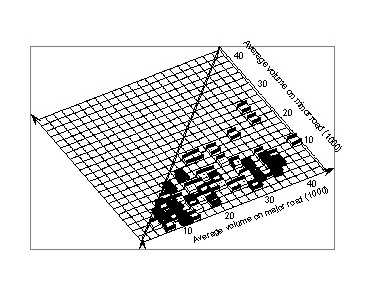Figure 73. Distribution of approach volumes of four–leg signalized urban intersetions in Minnesota. The width of the gridlines is proportional to the number of intersections in each cell.

Top

### 6.2 Smoothed crash counts

Figure 74 shows the smoothed values for the crash counts found in the data file, using a window of (4,000 x 4,000). There is a tendency for crash counts to increase roughly linearly with the minor volume, at least up to certain values. The relationship with the major volume, however, is more complicated. There is relatively little variation up to 16,000 vpd, and again from 22,000 vpd upward, except for some isolated points at high volumes. Between 16,000 vpd and 22,000 vpd, crash counts increase quite rapidly with the major volume.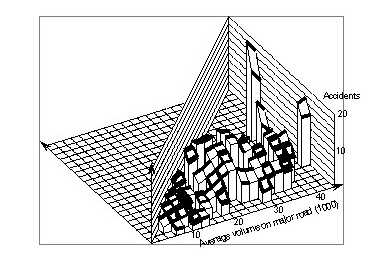Figure 74. Signialized four–leg urban intersections in Minnesota. Counts of accidents within intersections smoothed with a 4,000 x 4,000 window.

Figures 75 and 76 show this pattern more clearly. Figure 75 shows the surface cut off at a minor volume of 6,000 vpd, where the pattern, described above, is very pronounced. Figure 76 shows a cut-off at a major volume of 10,000 vpd. Here, the initial level part is no longer present, but there is a linear increase up to a major volume of 22,000 vpd, above which crash counts also remain stable.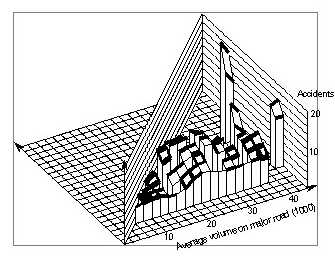Figure 75. The same surface as in Figure 74 but cut-off at a minor volume of 6,000.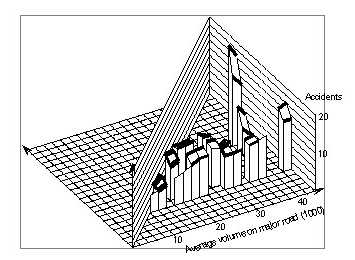Figure 76. The same surface as in Figure 74 but cut-off at a minor volume of 10,000.

While this pattern appears to be very clear, there is still a possibility that it is due to only a few intersections. Therefore, we also generated the surface using a larger smoothing window size of (8,000 x 4,000). The resulting surface is shown in Figure 77. The fairly steep increase between 16,000 vpd and 22,000 vpd has been smoothed out to a more uniform increase up to 24,000 vpd. Beyond that, however, crash counts still vary only slightly with the major volume, except for a few points with very high volumes.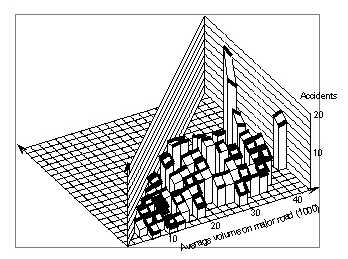Figure 77. Signalized four–leg urban intersections in Minnesota. Accident counts within intersections smoothed with a 8,000 x 4,000 window.

Top

### 6.3 An analytical model

Figure 77. Signalized four–leg urban intersections in Minnesota. Accident counts within intersections smoothed with a 8,000 x 4,000 window.

 z = 2.18*x0.205y0.388 (6-1) (0.63) (0.102)(0.090)

Figure 78 shows the surface representing this model. Like the smoothed surface, It shows only a weak increase of crash counts with major volume.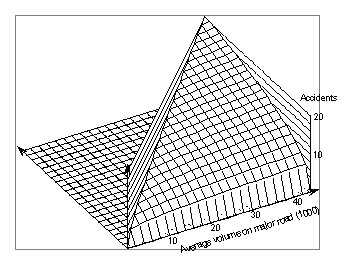Figure 78. Signalized four-leg urvan intersections in Minnestoa. Surface represents model (6-1) for within intersection accident counts.

Obviously, it cannot represent the different pattern for lower volumes. This becomes clear when Figure 79, which shows the surface cut–off at a minor volume of 6,000 vpd, is compared with Figure 75, which shows the actual surface cut–off at the same place. If Figure 80 is similarly compared with Figure 76, it is clear either that log–linear models are inadequate, or that intersections with low major volume differ from those with high major volume by some important feature that starts appearing at volumes between 16,000 vpd and 22,000 vpd.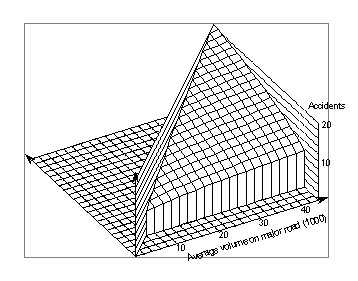Figure 79. The same surface as in Figure 78, but cut–off at y = 6,000.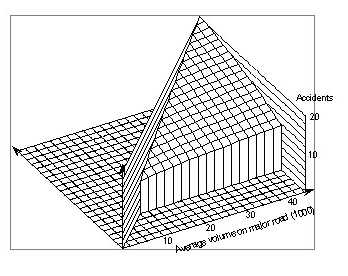Figure 80. The same surface as in Figure 78, but cut–off at y = 10,000.

Top

### 6.4 Crash types

Since total crash counts aggregate very different types of crashes, studying different crash types separately may provide insights that cannot be found in aggregate crash patterns. The simplest distinction between crash types is by location, that is, whether the crash occurred within the intersection proper or on an approach to the intersection.

Figures 81 and 82 show the smoothed relation of all crashes in the intersections proper and within 60 meters of the intersections. There is a general similarity between Figure 81 and Figure 74 which represents only crashes within the intersection proper.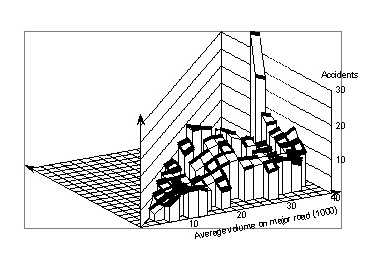Figure 81. Signalized four-leg urban intersections in Minnesota. Accident in the intersection and on the approaches withing 200=, smoothed with a 5,000 x 5,000 window.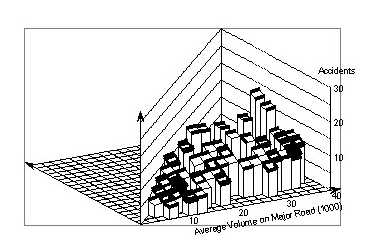Figure 82. Signalized four-leg urban intersections in Minnesota. All accident in the intersection and on the approaches within 60 meters, smoothed with a 15,000 x 10,000 window.

A closer look, however, shows some differences that are reflected in Figures 83 and 84, which show crashes that occurred on the approaches within 60 meters of the intersection. The less smoothed surface in Figure 83 shows no clear pattern. The more smoothed surface in Figure 84 shows a weak but steady increase with the major volume, and relatively little variation with the minor volume. This simple pattern is very different from the pattern seen in Figure 76, where the increase with the major volume is less consistent, but the increase with the minor volume is strong.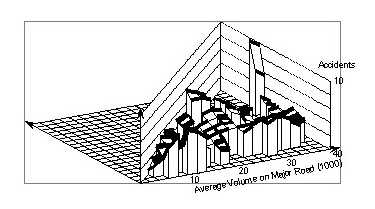Figure 83. Signalized four-leg urban intersections in Minnesota. All accidents on the approaches outside the intersection within 60 meters, smoothed with a 5,000 x 5,000 window.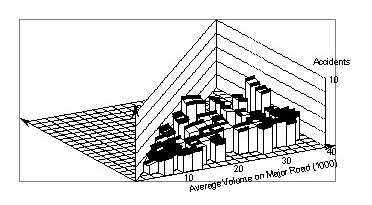Figure 84. Signalized four-leg urban intersections in Minnesota. All accidents on the approaches outside the intersection within 60 meters smoothed with a 10,000 x 10,000 window.

Even crashes within the intersection itself are not homogeneous. They can result from very different pre–crash situations and intended maneuvers. The occurrence of certain crash configurations depends on both the frequencies of the underlying maneuvers and on their inherent crash risk. One would expect that intersections with a high proportion of "risky" maneuvers also have higher crash counts.

The crash file contains some information on crash configuration. We excluded crashes characterized as "other" or "unknown." We also excluded crashes where the vehicle ran off the road, because they are not typical for an intersection. The other "typical intersection crashes" were grouped as follows:

• rear end
• left turn
• right turn
• angle
• other, consisting of side–swipes and head–on collisions

A number of intersections had no typical intersection crashes. Figure 85 shows the distribution of volumes for intersections with typical intersection crashes. A comparison with Figure 73 shows relatively few differences.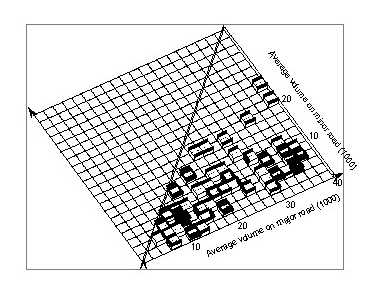Figure 85. Signalized four-leg urban intersections in Minnesota. Distribution of intersections with typical intersections accidents by volumes of the two roads.

Figures 86 and 87 show the surfaces for the typical intersection crashes. The less smoothed surface in Figure 86 shows some irregular variation, aside from a few unusually high cases. If we again disregard the few high–volume cases, the more smoothed surface in Figure 87, shows practically no variation with the major volumes and only little variation with the minor volumes. This is an interesting observation for which we do not have even a speculative explanation. Counts for specific crash types are even lower and, therefore, show more random variations. The proportions of the crash types tended to vary less and are therefore shown.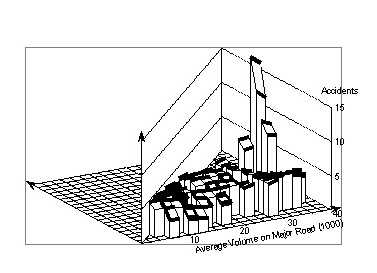Figure 86. Signalized four-leg urban intersections in Minnesota. Typical intersection accident, smoothed with a 10,000 x 5,000 window.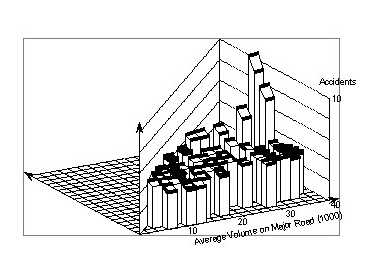Figure 87. Signalized four-leg urban intersections in Minnesota. Typical intersection accident within the intersection, smoothed with a 20,000 x 10,000 window.

Figure 88 shows this proportion of crashes involving left turns. This proportion tends to decrease with increasing major volumes, though not uniformly. The pattern changes in a systematic way with regard to minor volume. For low values of the major volume the proportion of left–turn crashes decreases with the minor volume, for medium volumes it stays nearly constant, and for high values of the major volume it increases with the minor volume. The surface pattern can be roughly characterized as a twisted sheet.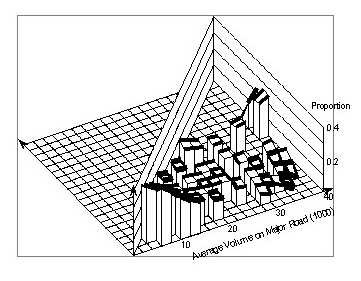Figure 88. Signalized four-leg urban intersections in Minnesota. Left-turn accidents within the intersection as proportion of typical intersection accidents, smoothed with a 10,000 x 15,000 window.

Figure 89 represents angle collisions. Again it is complicated but can be simply described as an elongated saddle.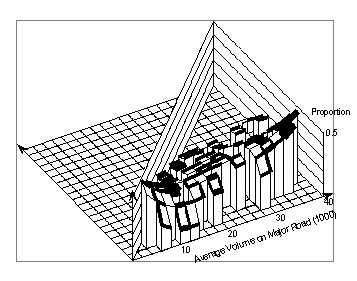Figure 89. Signalized four–leg urban intersections in Minnesota. Angle collisions within the intersection as proportion of typical intersection accidents, smoothed with a 10,000 x 15,000 window.

Figure 90 shows the surface for rear-end collisions, which is somewhat complementary to that for the angle collisions.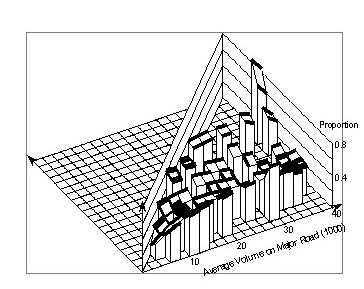Figure 90. Signalized four-leg urban intersections in Minnesota. rear–end collisions within the intersection as proportion of typical intersection accidents, smoothed with a 10,000 x 5,000 window.

A different pattern is seen for "other" collisions in Figure 91. However, there is a ridge, which is approximately at the same location as the ridge in Figure 89 and the trough in Figure 90. Together, these three figures suggest that around minor road volumes of 4,000 vpd to 6,000 vpd there is a change in the crash pattern. There were too few right–turn crashes to allow a meaningful representation by smoothing.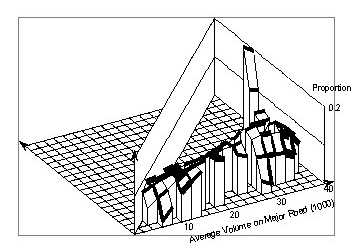Figure 91. Signalized four-leg urban intersections in Minnesota. Other collisions within the intersection as proportion of typical intersection accidents, smoothed with a 10,000 x 6,000 window.

Top

### 6.5 Relating proportions of crash types to number of intersection crashes

The idea that led to this analysis was that an intersection with a high proportion of left turns would also have a high proportion of left–turn crashes. Because of the high risk in left turns, such an intersection would have a higher crash count than otherwise similar intersections with few left turns. If this turned out to be the case, the proportion of left–turn crashes could be used as a proxy for exposure.

For the following analyses, we used only those intersections that had crashes of the selected types within the intersection and had a minor volume of less than 16,000 vpd, because the few intersections with greater volumes had much higher crash counts than others and showed only very weak relationships between volumes and crash counts. Fifty–seven intersections were selected.

As a first step, we looked at relationships between the number of crashes within the intersections and the proportions of the four crash types we distinguished. These data are plotted in Figures 91 to 95. Also shown are crash counts smoothed over the proportions. A common pattern is that the smoothed values are always low for proportions of 0 and 1, and are at their highest value near 0.25 to 0.3. Interpreted literally, this means that total crashes would be the highest if the four crash types were about equally present, that is, each accounted for about 25 percent of the total crashes. Furthermore, the total crashes would be lowest if all the crashes were of one type.

However, it is easy to see that this is a statistical artifact. Intersections with only one crash can have only the proportions 0 or 1 for each crash type. For instance, in Figure 91 there are eight intersections with one crash that have no angle collisions, and two that have one angle collision. For intersections with two crashes the proportions of 0, 0.5, and 1 are possible. In Figure 92 there are four intersections with no angle collisions, six with one, and one with two. More possibilities appear with increasing number of collisions. For the one intersection with 17 crashes, the proportions 0, 0.059, 0.118, ........0.941, and 1.0 are possible. Actually this intersection has proportions of 0.294 for angle collisions, 0.412 for left–turn collisions, 0.235 for rear–end collisions, and 0.059 for other collisions. It can be concluded from this observation that, if there were no relationships between crash counts and proportions of crashes, and the probabilities for the four types are equal, then most intersections with many crashes would have proportions near 0.25, and very few intersections, if any, would have proportions near 0 or 1. The lower the number of crashes, the more often intersections with proportions of 0 or 1 must appear, until finally intersections with one crash can have only zero or one crashes of each type.

A consequence of the above is that primarily intersections with low crash count are represented at the endpoints 0 and 1 and that the average crash counts at these points must be low. Other proportions can be realized only at intersections with more crashes and, therefore, for proportions between 0 and 1, the average crash count must be higher.

Correcting for this confounding factor is very difficult. However, it can be avoided if one looks at the graph "the other way," that is, by looking at proportions of crashes versus crash counts. Figures 96 to 99 show the data this way. For each crash count (intersections with the same crash count are combined) the proportions of crashes of one of the four types are shown, together with the range of one standard error. For proportion 0, a standard error of 0.04 was assumed.

The smoothed curves also are shown. They use a generalized Gaussian kernel and weight the points according to their standard errors. Figure 98, which shows the proportion of rear–end crashes, is the only figure that shows a potential relationship between total crashes and the proportion of crashes of one type. To explore this further, we used our original idea that the proportions of crash types might possibly explain deviations of individual intersection counts from the average surface for all intersections, which should be determined largely by volume effects. Therefore, we calculated the difference between the intersection crash count and the value from the smoothed surface for each of the 57 intersections. These differences are used in Figure 100. Comparing Figure 100 with Figure 98, which shows the smoothed surface for the actual crash counts, shows that although the two figures initially look very different, there are some similarities. A comparison with Figure 94 shows even greater similarities (note that abscissa and ordinates are exchanged). The clusters of data points that appear in Figure 94 are spread out, but not much. The reason is that the differences against the smoothed value do not differ much from the difference against the overall average, because the smoothed surface does not differ much from the overall average.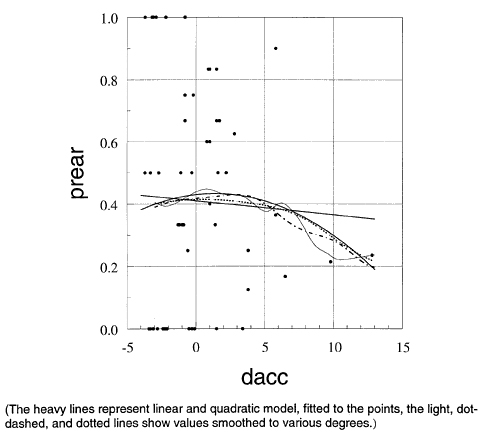Figure 100. Signalized four–leg urban intersections in Minnesota. Other collisions within the intersection as proportion of typical intersection accidents, smoothed with a 10,000 x 6,000 window.

The straight line shows a linear regression fitted to the data points. Its slope does not differ significantly from zero. This can be deceiving, since the linear specification of the model may be wrong. A quadratic regression fit gave the bold curved line. None of the coefficients, or both coefficients together, were significantly different from zero. Again, the quadratic specification may not be adequate. Smoothing with a wide window gave the broken line close to the quadratic model. Smoothing with an intermediate window gave the dash–dotted line, and smoothing with a narrow window gave the light solid line, which, aside from local variations, suggests a step somewhere between crash differences of 6 to 9.

The smoothed functions do not provide simple error estimates as regression models do. However, some estimates can be obtained. One approach utilizes the concept that each point of the smoothed curve is an estimated point of a local regression. The error of this estimate can be used as the error of the smoothed value. An objection to this approach is that both the proportion and the crash difference are random variables. The standard error estimates of regression analysis, however, assume that only the dependent variable is subject to random errors. An alternative, nonparametric approach is bootstrapping. One way to bootstrap is to fit a smooth model, calculate the residual of the data points against the model, repeatedly add random samples (with replacement) of the residuals to the smoothed values, and smooth these artificial values. If one repeats this often enough, one obtains a visual "confidence band" where only a certain specific percentage of the smoothed points lie outside this band. This approach, however, makes the same unrealistic assumption as the classical regression approach, i.e., that the values of the independent variables have no errors. This assumption can be avoided by a different, even simpler, application of the bootstrapping principle. Samples (with replacement) of 57 from the given 57 intersections are drawn repeatedly and a smoothed curve is fitted to each sample.

Figure 101 shows the results of 10 smoothing fits using the wide window. Beyond a crash difference of 7, the fit becomes very uncertain and often gives impossible negative proportions. Figure 102 shows the same curves, with the range of theFigure 101. Results of 10 smoothing fits using wide window.

ordinate between 0 and 1. Obviously, the smoothed relationship between the proportion of rear–end crashes and the crash difference is very uncertain, even where the proportions are not negative.

This high uncertainty is not a weakness of the smoothing technique. Rather, it is a result of the wide scatter of the data. This is confirmed in Figures 103 and 104. They show quadratic regressions fitted to 10 bootstrap samples of intersections. Except for one sample, the range of variability is as great as with the smoothed relations. If the comparison is limited to the range of feasible proportions between 0 and 1, the quadratic model is much less certain than the smoothed model, as shown in Figures 102 and 104. The reason is that the two data points with the highest values of crash difference are very "influential." To some extent smoothing can accommodate such influential points and reduce their influence on other parts of the curve. This is difficult with analytical models, and models with many parameters must be used.

Figure 105 shows the result of fitting linear regressions to the bootstrapped samples. Here the variability is much less than with the quadratic model. It should be noted that the envelope of the lines is very close to the typical 90 percent confidence "trumpet" derived by analytic models.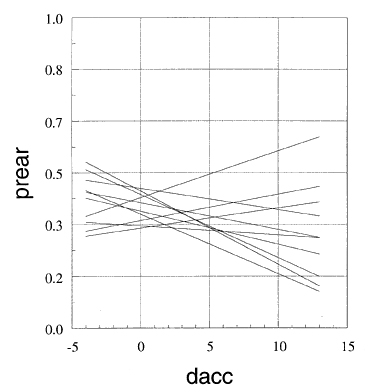Figure 105. Signalized for–leg intersections in Minnesota. Ten bootstrap replications of a quadratic model fit for the proportion of rear–end collisions.

Top

### 6.6 Conclusion

The overall conclusion is that only two intersections are responsible for the apparent, but not significant, relationship between the proportion of rear–end collisions and the crash difference. To determine whether such a relationship might be real, a much larger number of intersections would be needed. No relationship between their proportion and the deviation of crash counts from the smoothed model was apparent for the other crash types.

Top

FHWA-RD-98-096